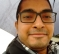## Difference between fft and pspectrum in MatlabStarted by 9 months ago3 replieslatest reply 9 months ago394 views
Hello!What is difference between using the fft() or pspectrum() command in Matlab on a time-series signal? My understanding is that the fft command computes the...

## Soft-output Hamming decoderStarted by 2 years ago1 replylatest reply 2 years ago157 views
Hello, I am implementing an SISO Hamming decoder in Matlab for (4,7) and (4,8) Hamming. The soft-input is coming from a soft demodulator, and the soft values are...

## Algorithm testing via simulation in Scilab/XcosStarted by 3 years ago11 replieslatest reply 3 years ago443 views
Hello,I have been developing a library of control blocks (PID controller, various filters etc.) in C programming language. My goal is to have basic building stones...

## Help on Noise Correlation MatrixStarted by 4 years ago10 replieslatest reply 4 years ago332 views
I was trying to create a Noise Cross Correlation Matrix in Matlab by using the following code:N = 100; % Number of Samplesr1 = rand(1,N) + 1i*rand(1,N);r2 = rand(1,N)...Started by 4 years ago26 replieslatest reply 4 years ago1323 views
Hi all,Suppose I have an input signal \( x[n] \) of length \( N \), an FIR filter \( h[n] \) of length \( M \), and a window function \( w[n] \) of length \( N...

## WLAN Demodulation in MATLABStarted by 5 years ago5 replieslatest reply 5 years ago259 views
I am using the WLAN Tool Box of #Matlab V2107b. A term named CSI for each data subcarrier is defined as CSI(i) = H(i) X conj(H(i)) + N(i)H(i) = channel estimate...

## How to downconvert a Complex RF signal in Matlab?Started by 5 years ago14 replieslatest reply 5 years ago2898 views
Hi All,I was trying to simulate a digital communication model with a complex rayleigh fading channel in Matlab.The Data is BPSK modulated and is multiplied with...

## Help on Demodulating an 8-PSK SignalStarted by 5 years ago21 replieslatest reply 5 years ago905 views
Hi,I am trying to simulate an 8-PSK communication model in Matlab with real sequence. I have generated the tx signal as shown in the attached figure using 8 different...

## Choice between Scilab and Python + numpyStarted by 6 years ago7 replieslatest reply 6 years ago4703 views
Hi everyone,I have a small bit of DSP to do related to work which has given me the oportunity to refresh prior DSP learning and expand it. I want to do this at home...

## #Matlab rcosfir functionStarted by 6 years ago4 replieslatest reply 6 years ago812 views
figure; plot(rcosfir(.2, 3, 12, 1/9600, 'sqrt') - rcosfir(.2, 3, 12, 1, 'sqrt'))The difference is negligible. Why does the 'T' parameter (symbol period) seem to...